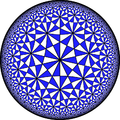# PerimeterThe perimeter of a shape is the distance around the sides of the shape. Calculating and distinguishing characteristics of shapes is an important concept in Geometry. Elementary school students should be familiar with both 2-D (square, triangle, rectangle, circle) and 3-D shapes (cube, sphere, cylinder, pyramid, cone). Kindergarteners and first graders should be able to identify the shape by name, whereas 2nd and 3rd graders should be able to calculate the perimeter, and 4th and 5th graders should be well versed in area and volume calculations.

In this activity, students will explore the concept of perimeter and how to calculate the perimeter of regular polygons.

Materials:

Measuring tape

White board and dry erase markers

Note: If your classroom is not perfectly square or rectangular, consider drawing a classroom layout, on the white board that is easy to calculate the perimeter. For more advanced students, challenge them to find the perimeter of their irregularly shaped classroom.

Instructions

1. Have the students measure the dimensions of the classroom (to the nearest foot), drawing a diagram of the classroom.
2. As the students measure the classroom, either have them recreate the classroom on paper, or on the white board in the front of the classroom.
3. Explain to the class that they are calculating the perimeter of the classroom. You may consider saying, “The perimeter are the edges of the classroom that we are measuring. The perimeter tells you the distance around the outside of a shape. The classroom is shaped like a rectangle (or a square), so when do you think it would be helpful to know the distance around the classroom? Yes, if we were going to decorate the classroom with a ribbon, we would need to know the length of the sides of the classroom. The perimeter is an easy calculation to find, just by adding the length of each side together.”
4. Have the students calculate the perimeter of the classroom using their diagrams.

You can extend this activity and encourage the students to measure a room in their home and determine the perimeter of the room. This can also be done measuring the perimeter of the outside playground at the school, or the perimeter of the school’s desks.

For more ideas about how to teach young students about perimeter and distance of shapes, visit: http://www.scholastic.com/teachers/top-teaching/2012/12/10-hands-strategies-teaching-area-and-perimeter and http://www.watchknowlearn.org/Video.aspx?VideoID=32320&CategoryID=3337.

or more fun and interesting Learning Math Games, you can visit us here:
http://www.math-lessons.ca/activities/FractionsBoard5.html
http://www.math-lessons.ca/timestables/times-tables.html
http://www.math-lessons.ca/activities/FractionsBoard4.html